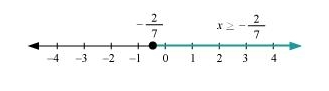# Solve the given inequality and show the graph of the solution on number line:

Question:

Solve the given inequality and show the graph of the solution on number line:  $\frac{x}{2} \geq \frac{(5 x-2)}{3}-\frac{(7 x-3)}{5}$

Solution:

$\frac{x}{2} \geq \frac{(5 x-2)}{3}-\frac{(7 x-3)}{5}$

$\Rightarrow \frac{x}{2} \geq \frac{5(5 x-2)-3(7 x-3)}{15}$

$\Rightarrow \frac{x}{2} \geq \frac{25 x-10-21 x+9}{15}$

$\Rightarrow \frac{x}{2} \geq \frac{4 x-1}{15}$$\Rightarrow 15 x \geq 2(4 x-1)$

$\Rightarrow 15 x \geq 8 x-2$

$\Rightarrow 15 x-8 x \geq 8 x-2-8 x$

$\Rightarrow 7 x \geq-2$

$\Rightarrow x \geq-\frac{2}{7}$

The graphical representation of the solutions of the given inequality is as follows.